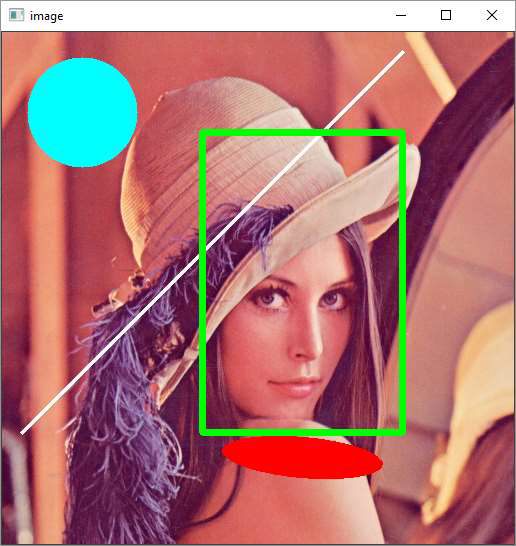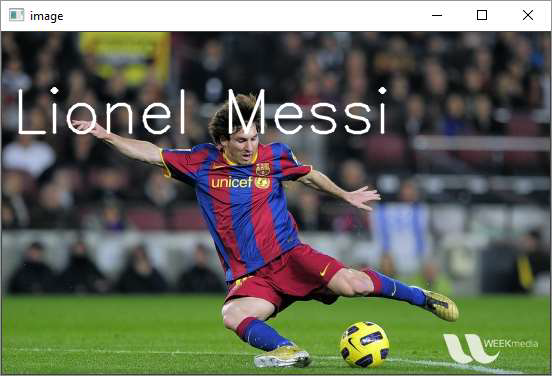# OpenCV Python - Draw Shapes and Text

In this chapter, we will learn how to draw shapes and text on images with the help of OpenCV-Python. Let us begin by understanding about drawing shapes on images.

## Draw Shapes on Images

We need to understand the required functions in OpenCV-Python, which help us to draw the shapes on images.

### Functions

The OpenCV-Python package (referred as cv2) contains the following functions to draw the respective shapes.

Function Description Command
cv2.line() Draws a line segment connecting two points. cv2.line(img, pt1, pt2, color, thickness)
cv2.circle() Draws a circle of given radius at given point as center cv2.circle(img, center, radius, color, thickness)
cv2.rectangle Draws a rectangle with given points as top-left and bottom-right. cv2.rectangle(img, pt1, pt2, color, thickness)
cv2.ellipse() Draws a simple or thick elliptic arc or fills an ellipse sector. cv2.ellipse(img, center, axes, angle, startAngle, endAngle, color, thickness)

### Parameters

The common parameters to the above functions are as follows −

Sr.No. Function & Description
1

img

The image where you want to draw the shapes

2

color

Color of the shape. for BGR, pass it as a tuple. For grayscale, just pass the scalar value.

3

thickness

Thickness of the line or circle etc. If -1 is passed for closed figures like circles, it will fill the shape.

4

lineType

Type of line, whether 8-connected, anti-aliased line etc.

### Example

Following example shows how the shapes are drawn on top of an image. The program for the same is given below −

import numpy as np
import cv2
cv2.line(img,(20,400),(400,20),(255,255,255),3)
cv2.rectangle(img,(200,100),(400,400),(0,255,0),5)
cv2.circle(img,(80,80), 55, (255,255,0), -1)
cv2.ellipse(img, (300,425), (80, 20), 5, 0, 360, (0,0,255), -1)
cv2.imshow('image',img)
cv2.waitKey(0)
cv2.destroyAllWindows()


### Output## Draw Text

The cv2.putText() function is provided to write a text on the image. The command for the same is as follows −

img, text, org, fontFace, fontScale, color, thickness)


### Fonts

OpenCV supports the following fonts −

Font Name Font Size
FONT_HERSHEY_SIMPLEX 0
FONT_HERSHEY_PLAIN 1
FONT_HERSHEY_DUPLEX 2
FONT_HERSHEY_COMPLEX 3
FONT_HERSHEY_TRIPLEX 4
FONT_HERSHEY_COMPLEX_SMALL 5
FONT_HERSHEY_SCRIPT_SIMPLEX 6
FONT_HERSHEY_SCRIPT_COMPLEX 7
FONT_ITALIC 16

### Example

Following program adds a text caption to a photograph showing Lionel Messi, the famous footballer.

import numpy as np
import cv2